# Laminated Plates with OrientationThe purpose of this test is to check laminated plates with orthotropic materials using an angle of orientation.
You will use 2D meshes.Reference:

3D model with parabolic hexahedron elements (HE20), computed with CATIA.

##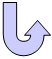Specifications

### Geometry Specifications

 Angle: plate 1 = 90 deg plate 2 = 30 deg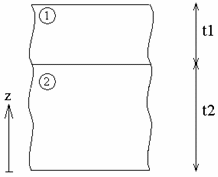a = 0.1 m b = 0.1 m t1 = 0.0002 m t2 = 0.0004 m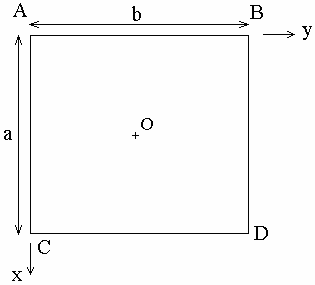### Analysis Specifications

 Material 1: Young Modulus (material):E1 = 341.6 x 106 Pa E2 = 179.3 x 106 Pa Poisson's Ratio: ν12 = 0.44  Shearing coefficient G12 = 100 x 106 Pa G23 = 60.8 x 106 Pa G13 = 101.5 x 106 Pa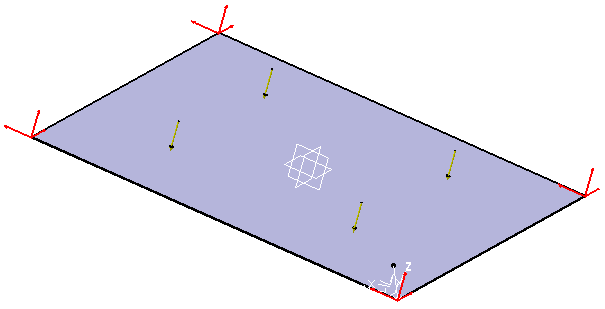Material 2: Young Modulus (material):E1 = 34.16 x 106 Pa E2 = 17.93 x 106 Pa Poisson's Ratio: ν12 = 0.44  Shearing coefficient G12 = 10 x 106 Pa G23 = 6.08 x 106 Pa G13 = 10.15 x 106 Pa Mesh Specifications: In both cases, mesh size: 2 mm 2D mesh with linear quadrangle elements (QD4) 3D mesh with parabolic hexahedron elements (HE20) Restraints (User defined): On AB and CD: translation along Y and Z On AC and BD: translation along X and Z Loads: Surface force density: FZ = -10 N/m2

##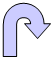Results

The following results correspond to:

• The displacement of O along Z (w)
• The restraint on O in the center of plate 2 (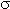xx)
 3D model (Reference) 2D model Normalized results w [mm] -3.47697 -3.47213 0.9986xx [mm] 30358.523 30217.189 0.9953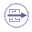To Perform the Test:

The laminated_plates.CATAnalysis document presents a complete analysis of this case.

To compute the case, proceed as follow:

1. Open the CATAnalysis document.

2. Compute the case in the Generative Structural Analysis workbench.

3. Create local sensors (Stress tensor and Displacement vector).